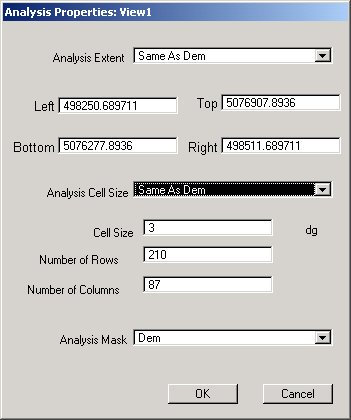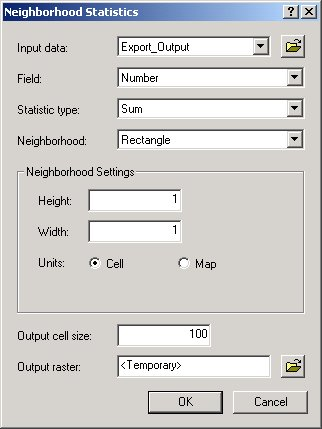English

# How To: Find the number of points in a grid using Spatial Analyst

## Summary

The following steps demonstrate how to find the number of points that fall within each cell of a grid.

## Procedure

Requires:
Grid with the cell size desired for analysis.
Point theme with a column that has the number 1 as its attribute for every record.

• For ArcView 3.x
1. Select Analysis > Properties to call up the 'Analysis Properties' dialog box.
2. Select the Grid as the Analysis Extent, the Analysis Cell Size, and the Analysis Mask.3. Click OK
4. Activate the point theme.
5. Select Analysis > Neighborhood Statistics to call up the 'Neighborhood Statistics' dialog box.
`    a. Select the Field:  The one that contains the value of 1 for each record.    b. Select the Statistic:  Sum    c. Select the Neighborhood: Rectangle    d. Width: 1    e. Height: 1    f. Select Units: Cell`• For ArcView 8.x
1. Select Spatial Analyst > Options to call up the 'Options' dialog box.
2. Select the grid as the Analysis extent, the Analysis cell size and the Analysis mask.
3. Click OK
4. Activate the point theme.
5. Select Spatial Analyst > Neighborhood Statistics to call up the 'Neighborhood Statistics' dialog box.
`    a. Select the Input data:  The point theme.    b. Select the Field:  The one that contains the value of 1 for each record.    c. Select the Statistic type:  Sum    d. Select the Neighborhood: Rectangle    g. Height: 1    f. Width: 1    g. Select Units: Cell``Note:Verify that the 'Output cell size' matches that of the input theme of choice.`